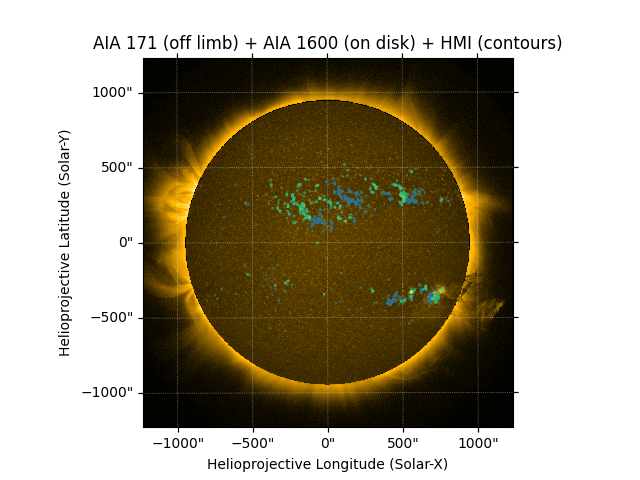# Combining off-limb and disk maps¶

This example combines creating a composite plot with masking out the solar disk. The purpose is to introduce users to the plotting process required to overlay multiple maps that have been modified with other `sunpy` functions. The resulting plot in this tutorial shows information on the upper photosphere, quiet corona, and magnetogram contours.

```import matplotlib.pyplot as plt

import astropy.units as u

import sunpy.data.sample
from sunpy.map import Map
from sunpy.map.maputils import all_coordinates_from_map, coordinate_is_on_solar_disk
```

Let’s import sample data representing the three types of data we want to overlay: AIA 1600 (upper photosphere), AIA 171 (quiet corona), and HMI data (magnetic fields in the photosphere).

```aia_1600 = Map(sunpy.data.sample.AIA_1600_IMAGE)
aia_171 = Map(sunpy.data.sample.AIA_171_IMAGE)
hmi = Map(sunpy.data.sample.HMI_LOS_IMAGE)
```

Next, let’s mask out the solar disk of the AIA 171 image since we will be overlaying the AIA 1600 photosphere.

```hpc_coords = all_coordinates_from_map(aia_171)
colormap = aia_171.cmap.copy()

```

Before we plot the composite image, let’s define the contour levels that will be applied to the HMI image. Also, grab the AIA 171 colormap to use with the AIA 1600 image to better match the images.

```levels = [-1000, -500, -250, 250, 500, 1000]*u.G
aia171_cmap = plt.get_cmap('sdoaia171')
```

Now, create the plot by overlaying the maps and contours. Note that `clip_interval` is being defined to highlight the coronal features of the scaled AIA 171 map and the AIA 1600 map is autoaligned to the AIA 171 WCS Axes.

```fig = plt.figure()